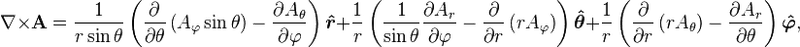The curl of a vector

Homework Statement

Evaluate ∇ x $\overline{F}$, with $\overline{F}$($\overline{r}$) = $\overline{r}$lnr, where $\overline{r}$ = (x; y; z) is the position vector and r is the modulus of the position vector.

Homework Equations

The curl of a vector

The Attempt at a Solution

I recognise I am evaluating the curl of the Vector F, however when taking the derivatives of the x, y and z components respectively. I'm not sure if it should be as follows. (showing the first bit of the problem)

($∂F(z)/∂y$ - $∂F(y)/∂Z$), ...

which becomes

(∂$\overline{r}$(z)lnr/∂y) - (∂$\overline{r}$(y)lnr/∂Z),

Im not sure if this is right and if I need to include the modulus of the vector r when differentiating $\overline{r}$lnr with respect to y or z.

ShayanJ
Gold Member
The way you did it is only applicable when the vector field is in Cartesian coordinates.But your vector field is in spherical coordinates and so its curl should be evaluated using the following formula:Im sorry, how is it in spherical coordinates? my vector is given with a position vector in (x,y,z), isn't that Cartesian?

vanhees71
Gold Member
2021 Award
Since the curl is a vector operator you can calculate in any coordinates you like. Here it's easier to use spherical coordinates then Cartesian ones, because in spherical coordinates your vector field (it's a curl of a vector field not simply a vector!) reads
$$\vec{V}(\vec{r})=r \hat{r} \ln r=A_r(r) \hat{r}.$$
You have to evaluate less derivatives than in Cartesian coordinates, but of course, you'll get the same result at the end.

I see. But i'm a little confused what V and A represent? I acknowledge you have used the unit vector multiplied by the magnitude of the vector r, as this is the same as the vector itself; but how am I suppose to obtain a value for the unit vector so I can use it?

pasmith
Homework Helper
I see. But i'm a little confused what V and A represent? I acknowledge you have used the unit vector multiplied by the magnitude of the vector r, as this is the same as the vector itself; but how am I suppose to obtain a value for the unit vector so I can use it?

$$\hat r = \frac{1}{\sqrt{x^2 + y^2 + z^2}}(x \hat i + y \hat j + z \hat k)$$

Thanks, but what do V and A represent?

Since the curl is a vector operator you can calculate in any coordinates you like. Here it's easier to use spherical coordinates then Cartesian ones, because in spherical coordinates your vector field (it's a curl of a vector field not simply a vector!) reads
$$\vec{V}(\vec{r})=r \hat{r} \ln r=A_r(r) \hat{r}.$$
You have to evaluate less derivatives than in Cartesian coordinates, but of course, you'll get the same result at the end.

And in the above, doesn't the r$\hat{r}$ just become (x$\hat{i}$+y$\hat{j}$+z$\hat{k}$)

vanhees71
I should have written $V_r$, and it's the radial component of the vector field. Again: It's of course the very same field in spherical coordinates as you've written in Cartesian coordinates. It's really easier to use spherical coordinates here.